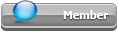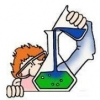## Featured Articles

Check out the latest featured articles.## File Library0# Heat Exchangers, Zone Analysis In Excel With Prode

heat exchanger zone analysis simulation thermophysical properties prode

5 replies to this topic
|

### #1Alpe

Alpe

Brand New Member

•• Members
•• 9 posts

Posted 20 August 2018 - 08:16 AM

I need to calculate vapor and liquid flows plus thermo physical properties (density, heating value, viscosity , thermal conductivity) for both vapor and liquid in different zones (zone analysis) of heat exchangers,

I know I can simulate heat exchangers in Excel with Prode Properties solving flash operations but how can I calculate vapor and liquid flows ?

Prode gives phase fractions (vapor, liquid, solid) not the flows,

can you provide a working example ? (with VBA or Excel macros)

Thanks !

### #2PaoloPemi

PaoloPemi

Gold Member

•• Members
•• 496 posts

Posted 20 August 2018 - 09:53 AM

there was a Excel page showing how to calculate properties in different zones I attach a copy, you can examine the VBA code for details,

Prode Properties can export flows or you can calculate from (molar) phase fractions and molar weights of different fractions, see this VBA example

PF = StrLf(Stream)
If (PF > 0.000001) Then ' Liquid present ?
mw = StrLMw(Stream)
Cells(rpt + I, 6) = W * PF * mw / mwm ' Liquid Flow

and so on...

you can calculate mixture properties (density, viscosity, thermal conductivity etc.) in different ways,

Prode Properties exposes methods StrGD, StrLD etc. (there are about 300 methods...)

in this Excel VBA example the procedure calculates vapor properties as

Cells(rpt + I, 14) = StrGMw(Stream)
Cells(rpt + I, 15) = StrGCp(Stream)
Cells(rpt + I, 16) = StrZv(Stream)
Cells(rpt + I, 17) = StrGD(Stream)
Cells(rpt + I, 18) = StrGV(Stream)
Cells(rpt + I, 19) = StrGC(Stream)

of course different variants are possible (many methods available),

you can solve many different Flash Operations with Prode Properties, in this case (heat exchanger simulation) the procedure solves a operation with specified P and H but different alternatives are possible### #3Alpe

Alpe

Brand New Member

•• Members
•• 9 posts

Posted 20 August 2018 - 10:43 AM

thanks,

### #4PaoloPemi

PaoloPemi

Gold Member

•• Members
•• 496 posts

Posted 20 August 2018 - 01:12 PM

StrLf(Stream) returns the total liquid fraction (with two liquid phases you obtain the total)

StrPf(Stream,Ph) returns the molar fraction of phase Ph which can be vapor, liquid or solid / hydrate ,

it depends from application...

### #5mgerian

mgerian

Brand New Member

•• Members
•• 5 posts

Posted 21 August 2018 - 08:33 AM

thanks Paolo for the Excel VBA example

### #6chemdoc

chemdoc

Junior Member

•• Members
•• 26 posts

Posted 26 September 2018 - 07:27 AM

thanks !